# Multiplying Mixed NumbersExample 1: Nina's garden is 4 and 2/3 feet long and 1 and 1/8 feet wide. What is the area of the garden?

Analysis: We will multiply these mixed numbers in order to solve this problem.

Solution: First we will convert each mixed number to an improper fraction. Then we can multiply.

Step 1:Step 2: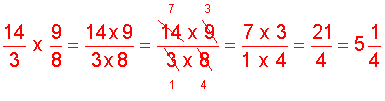Answer: The area of Nina's garden is 5 and 1/4 sq ft.

Example 2: Multiply.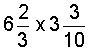Analysis: First convert each mixed number to an improper fraction. Then multiply.

Step 1: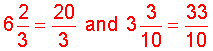Step 2: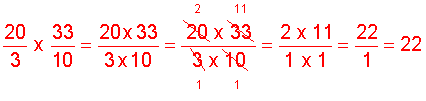The following is the procedure for multiplying mixed numbers..

Procedure: To multiply mixed numbers, first convert each mixed number to an improper fraction, then multiply. Simplify your result, if necessary.

Let's look at some examples using this procedure.

Example 3: Multiply.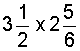Step 1:Step 2: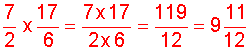There are no common factors to divide out.

Example 4: Multiply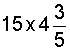Analysis: First, convert the whole number and the mixed number to an improper fraction. Then multiply.

Step 1: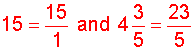Step 2:Now that we know how to multiply fractions, we do not need to show each and every part of the process below in our examples.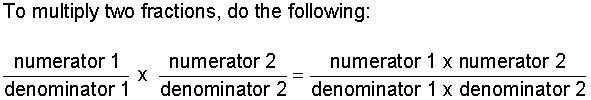Example 5: Multiply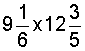Step 1:Step 2: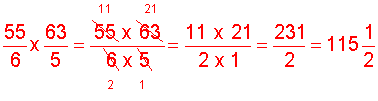Example 6: Multiply.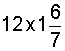Solution: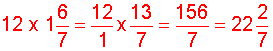There are no common factors to divide out.

Example 7: Multiply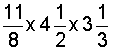Solution:Summary: To multiply mixed numbers, first convert each mixed number to an improper fraction, then multiply. Simplify your result, if necessary.

### Exercises

Directions: In each exercise below, multiply the fractions by dividing out common factors. Be sure to simplify your result, if necessary. Click once in an ANSWER BOX and type in your answer; then click ENTER. After you click ENTER, a message will appear in the RESULTS BOX to indicate whether your answer is correct or incorrect. To start over, click CLEAR.

Note: To write the mixed number four and two-thirds, enter 4, a space, and then 2/3 into the form.

 1.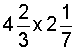ANSWER BOX:   RESULTS BOX:
 2.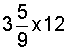ANSWER BOX:   RESULTS BOX:
 3.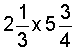ANSWER BOX:   RESULTS BOX:
 4.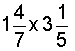ANSWER BOX:   RESULTS BOX:
 5.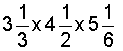ANSWER BOX:     RESULTS BOX:
 Lessons on Multiplying and Dividing Fractions and Mixed Numbers 1. Multiplying Fractions 2. Multiplying Fractions by Cancelling Common Factors 3. Multiplying Mixed Numbers 4. Reciprocals 5. Dividing Fractions 6. Dividing Mixed Numbers 6. Solving Word Problems 7. Practice Exercises 8. Challenge Exercises 9. Solutions